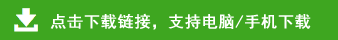# 苏教版二年级数学上册第一单元测试卷及答案

一、直接写出得数。(12分)

7＋66 =       54－9=         43＋30 =         46＋6－30=

65＋9 =       82－40=        27＋7 =          38＋4＋7 =

32－8 =       40＋28 =       56＋9 =          67－40＋21 =

19＋58－62=      90－45－27=

29＋35＋9=      75－46＋31=